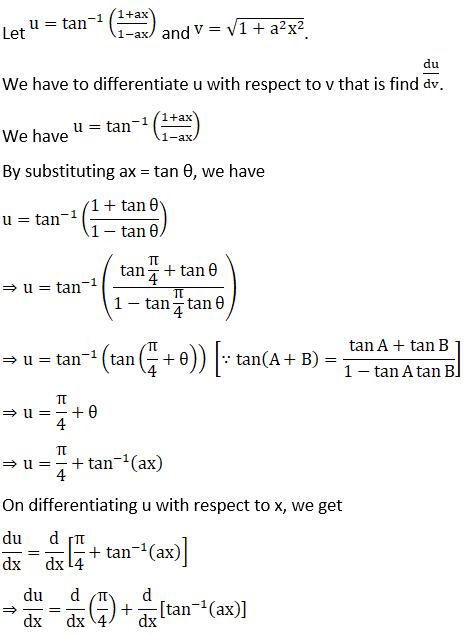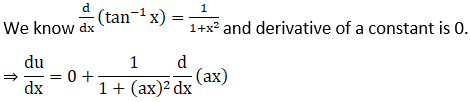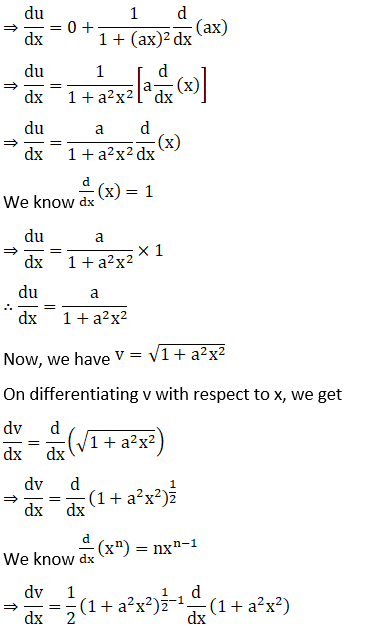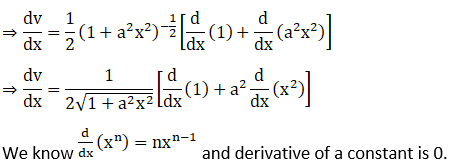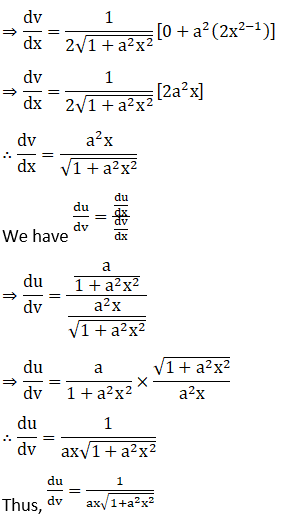# RD Sharma Solutions for Class 12 Maths Exercise 11.8 Chapter 11 Differentiation

RD Sharma Solutions for Class 12 Maths Exercise 11.8 Chapter 11 Differentiation is provided here. This exercise can be used as a model by the students to improve their conceptual knowledge and understand the different ways used to solve problems. Differentiation of a function with respect to another function is the main concept covered under this exercise.

These RD Sharma Solutions are formulated by BYJU’S experts in Maths to increase students’ confidence, which plays a crucial role in their board exams. The PDF of RD Sharma Solutions for Class 12 Maths Chapter 11 Exercise 11.8 can be easily viewed and downloaded from the given links.

## RD Sharma Solutions for Class 12 Chapter 11 Exercise 11.8

### Access other exercises of RD Sharma Solutions for Class 12 Chapter 11 – Differentiation

Exercise 11.1 Solutions

Exercise 11.2 Solutions

Exercise 11.3 Solutions

Exercise 11.4 Solutions

Exercise 11.5 Solutions

Exercise 11.6 Solutions

Exercise 11.7 Solutions

### Access answers to Maths RD Sharma Solutions For Class 12 Chapter 11 – Differentiation Exercise 11.8

Exercise 11.8 Page No: 11.112

1. Differentiate x2 with respect to x3.

Solution: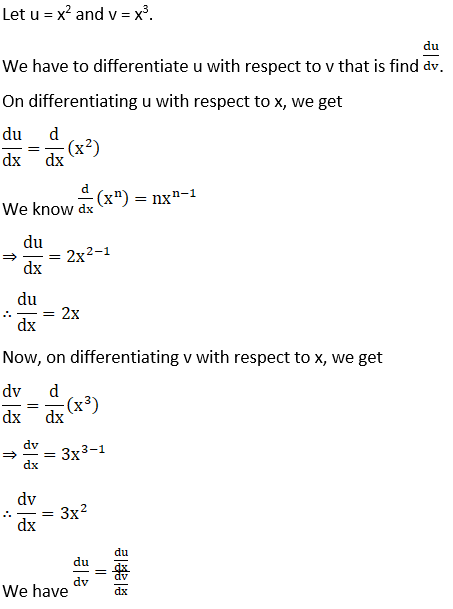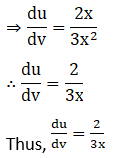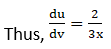2. Differentiate log (1 +x2) with respect to tan-1 x.

Solution: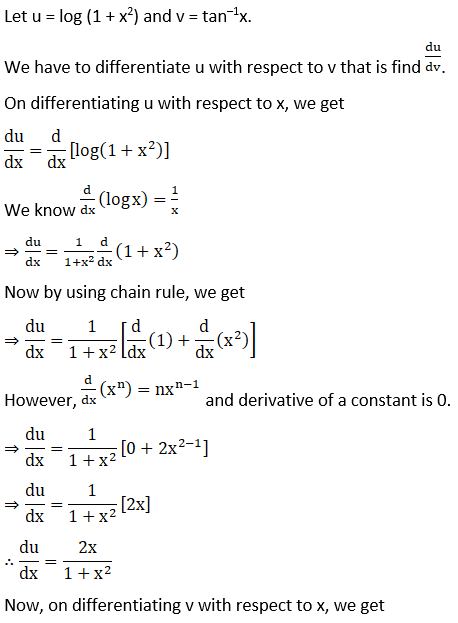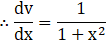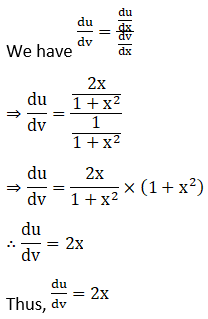3. Differentiate (log x)x with respect to log x.

Solution: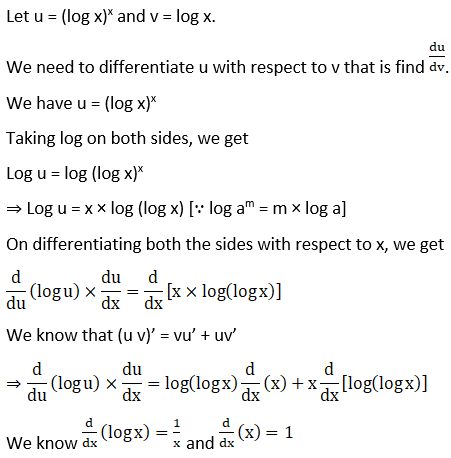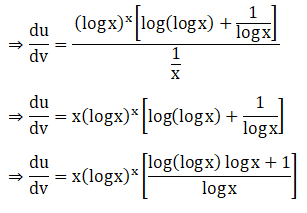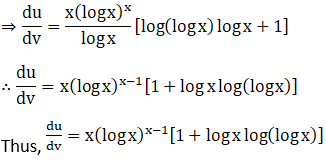4. Differentiate sin-1 √ (1-x2) with respect to cos-1x, if

(i) x ∈ (0, 1)

(ii) x ∈ (-1, 0)

Solution:

(i) Given sin-1 √ (1-x2)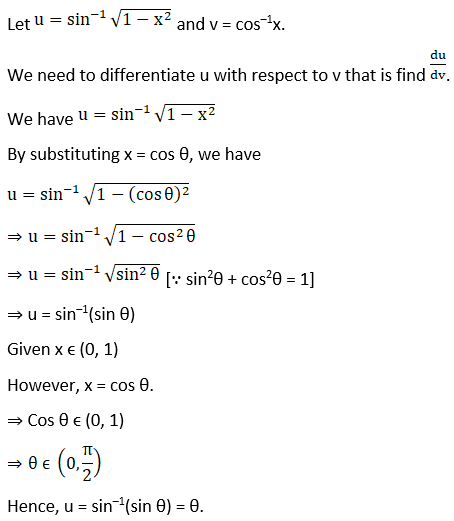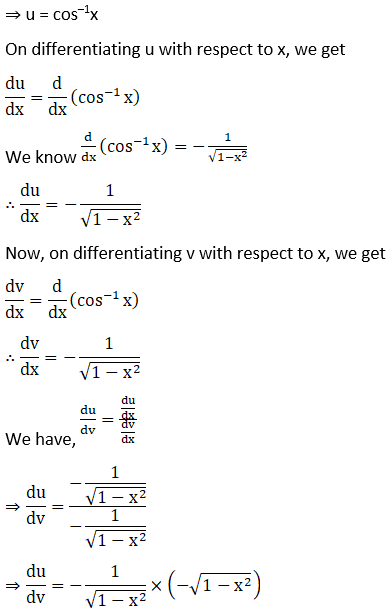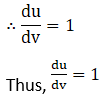(ii) Given sin-1 √ (1-x2)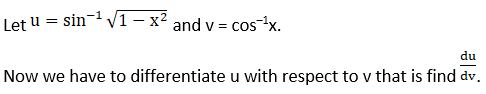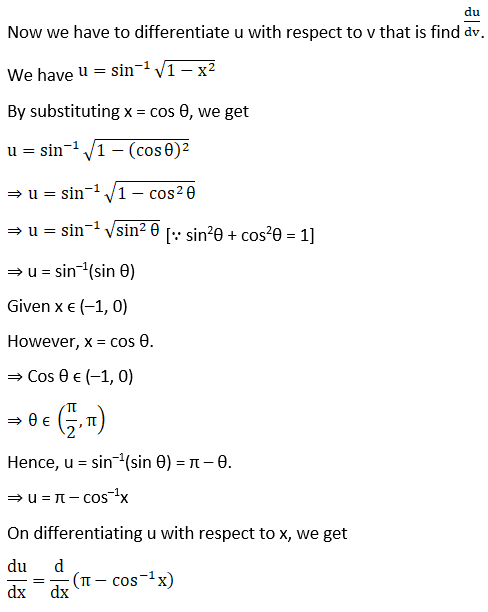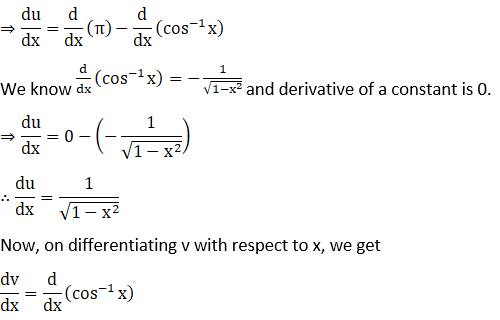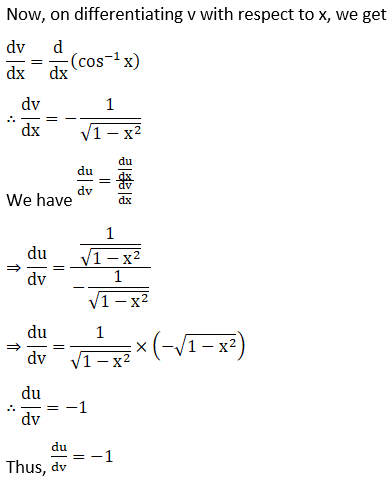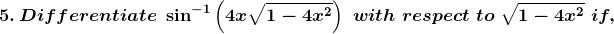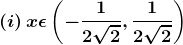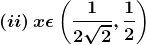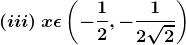Solution:

(i) Let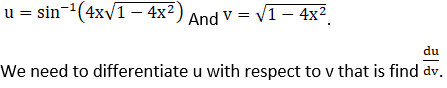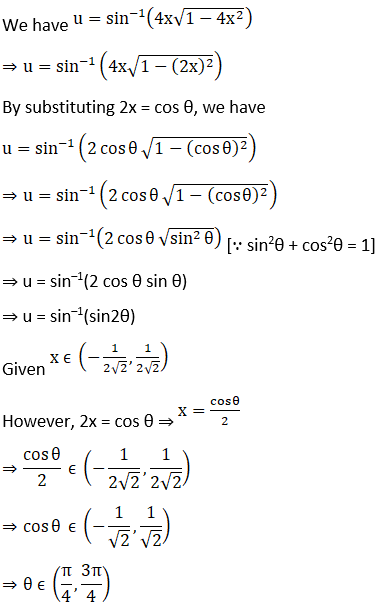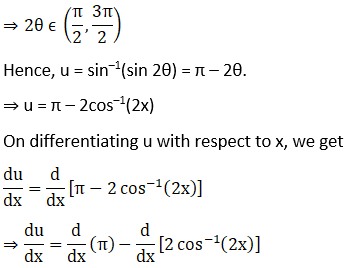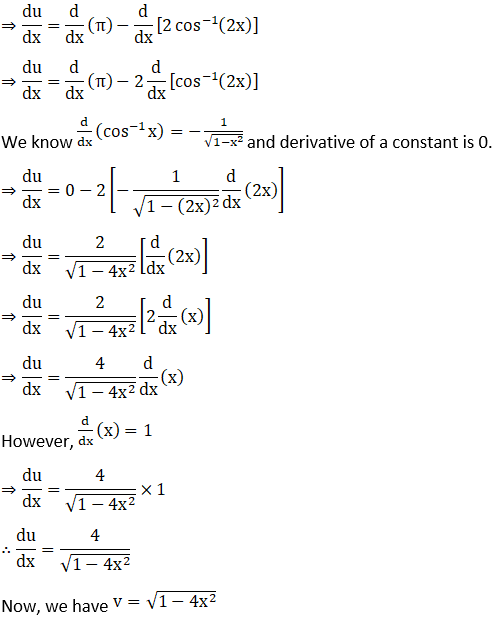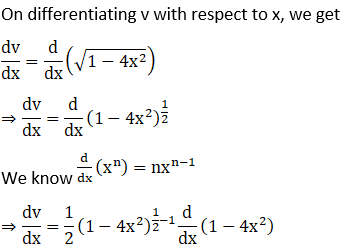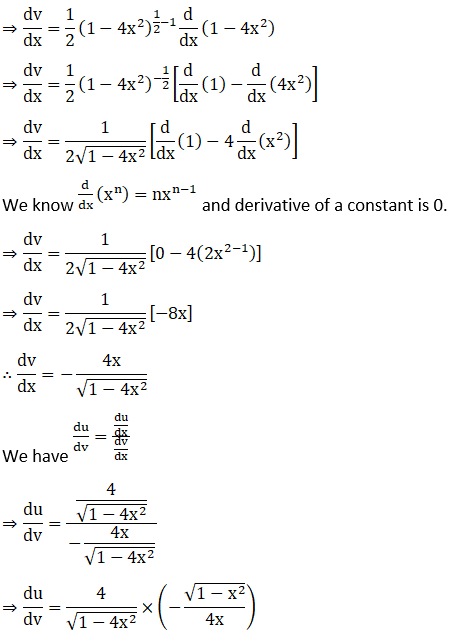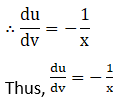(ii) Let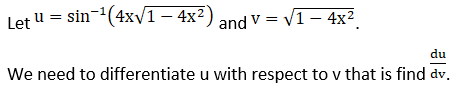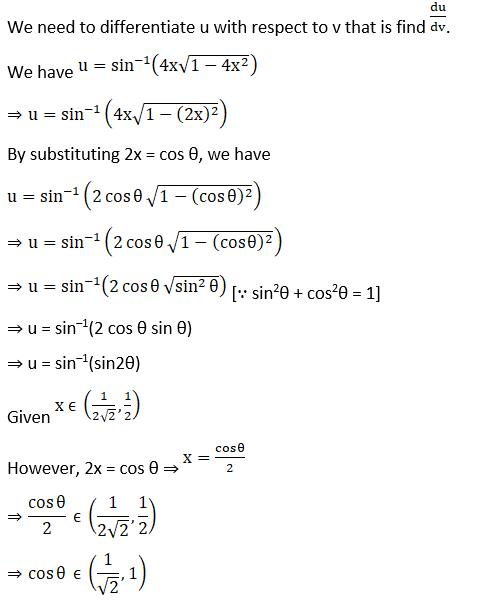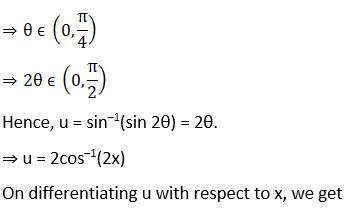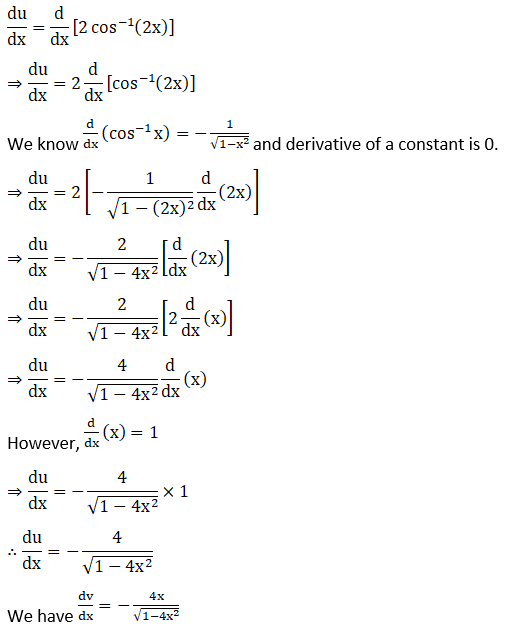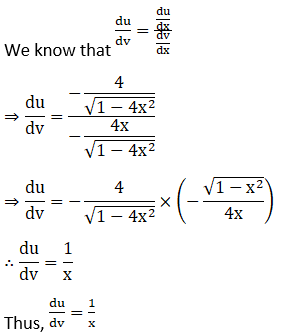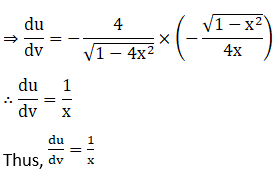(iii) Let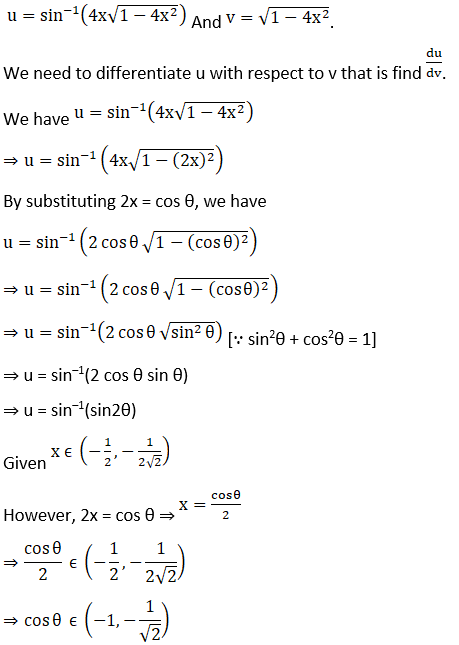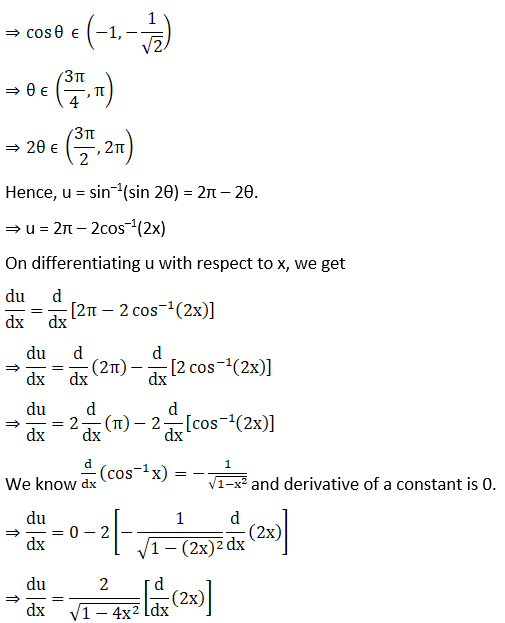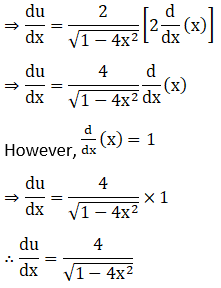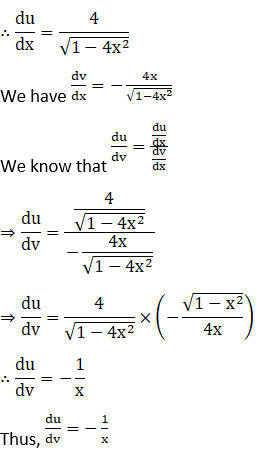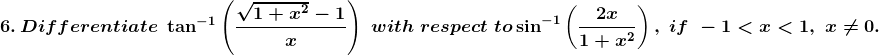Solution: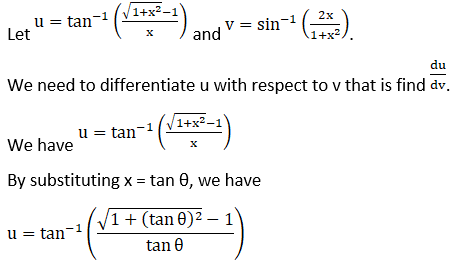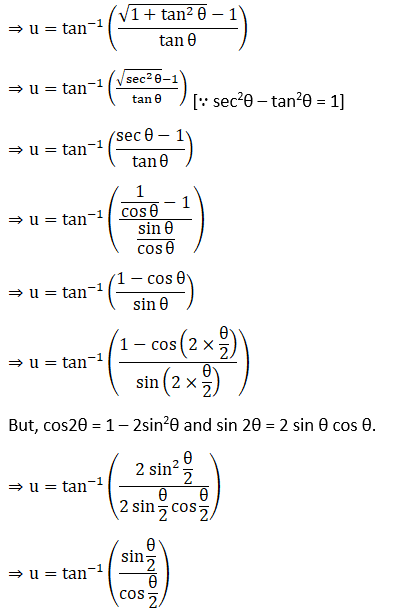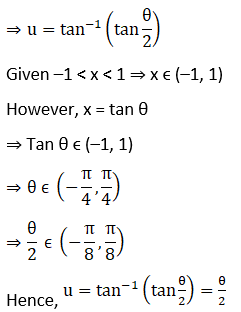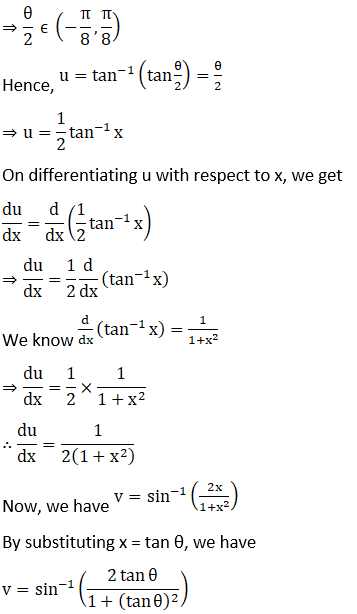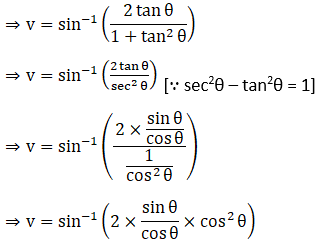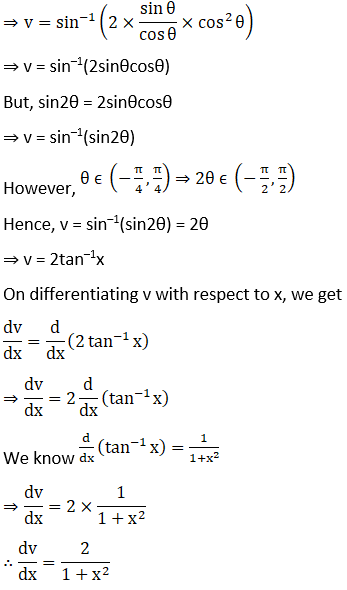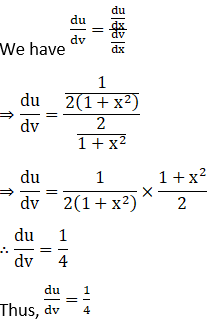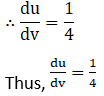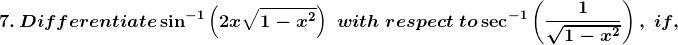(i) x ∈ (0, 1/ √2)

(ii) x ∈ (1/√2, 1)

Solution:

(i) Let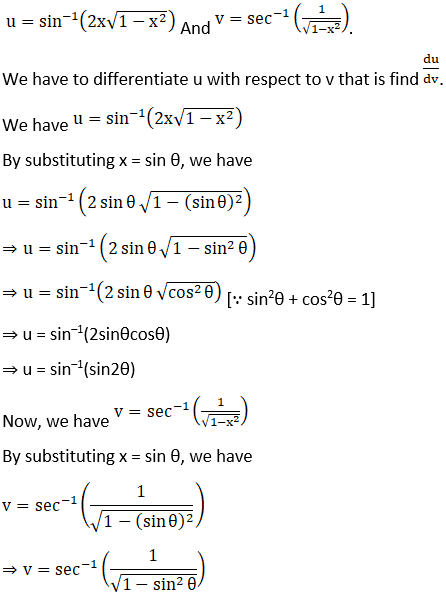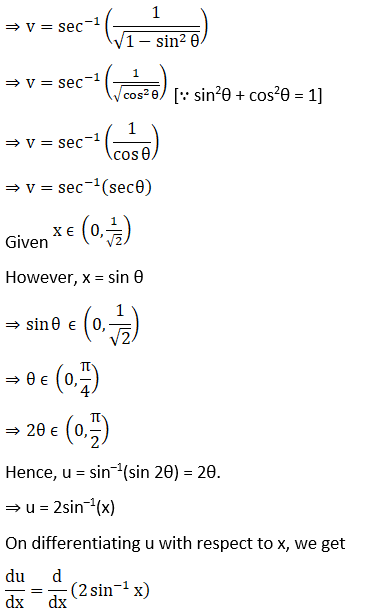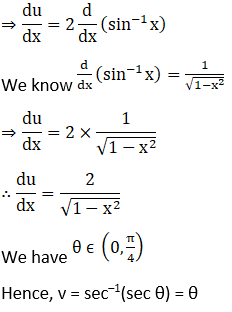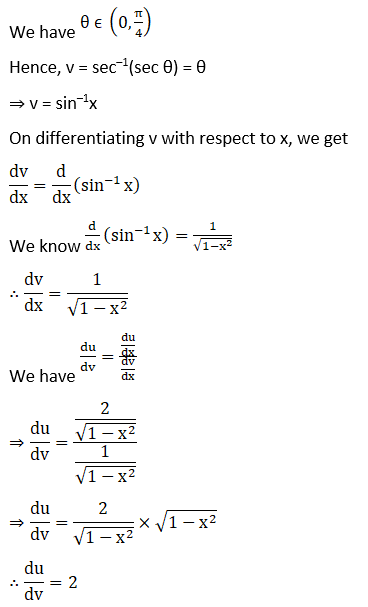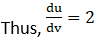(ii) Let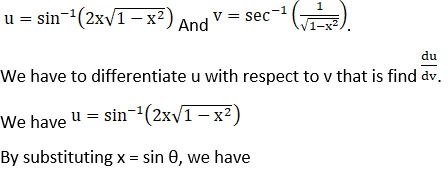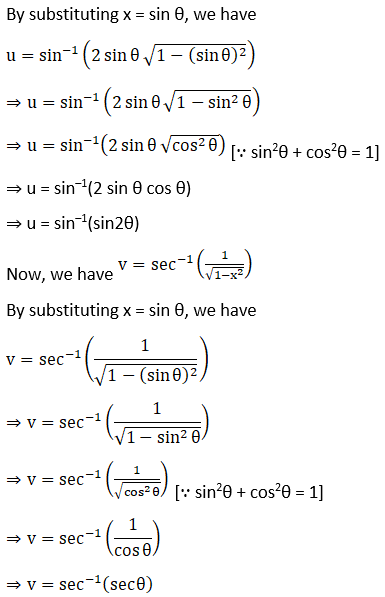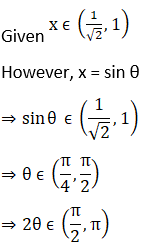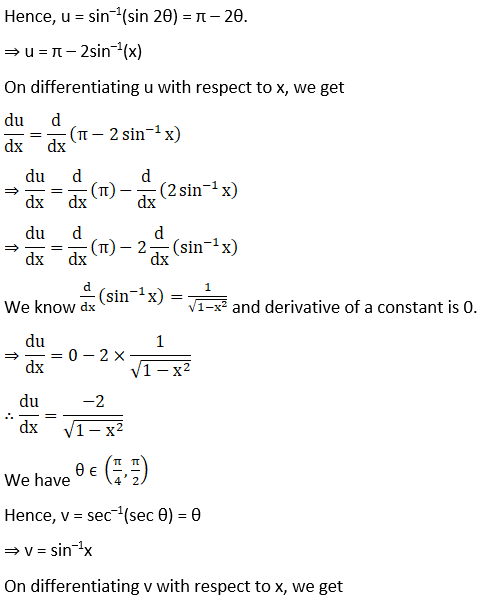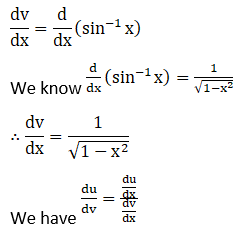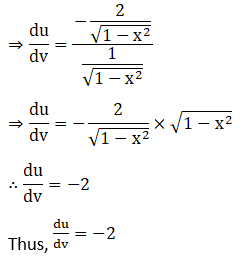8. Differentiate (cos x)sin x with respect to (sin x)cos x.

Solution: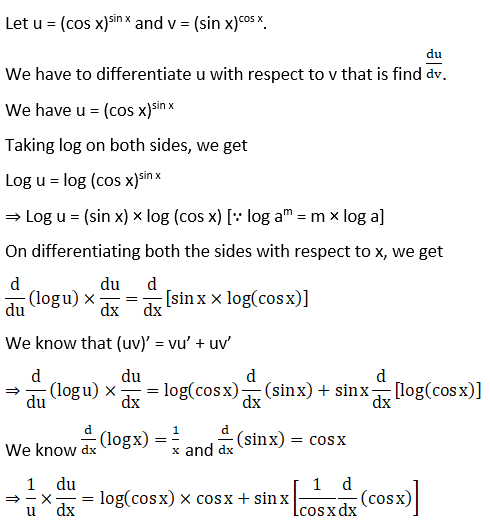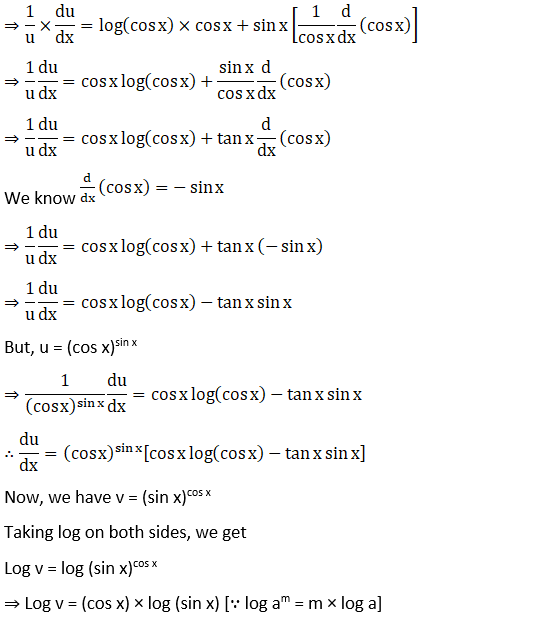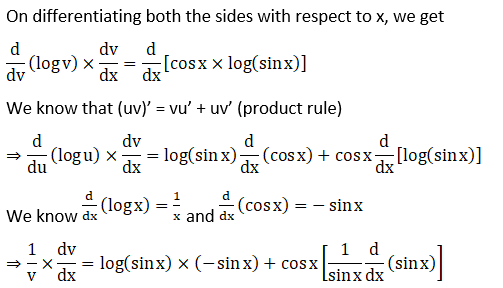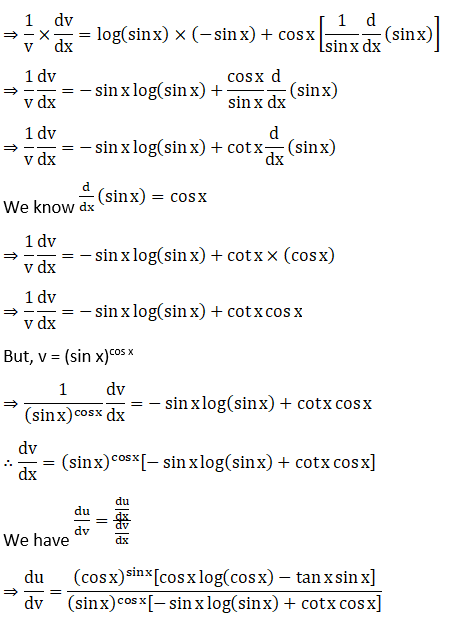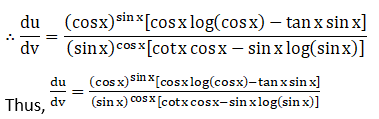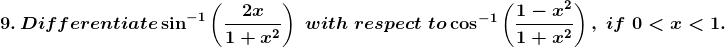Solution: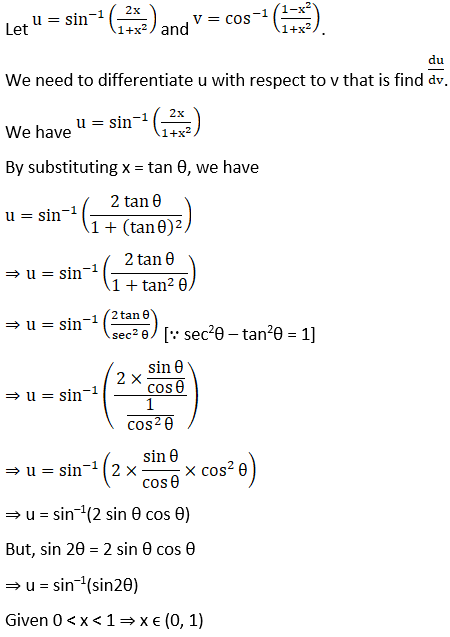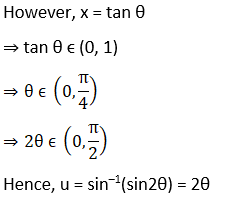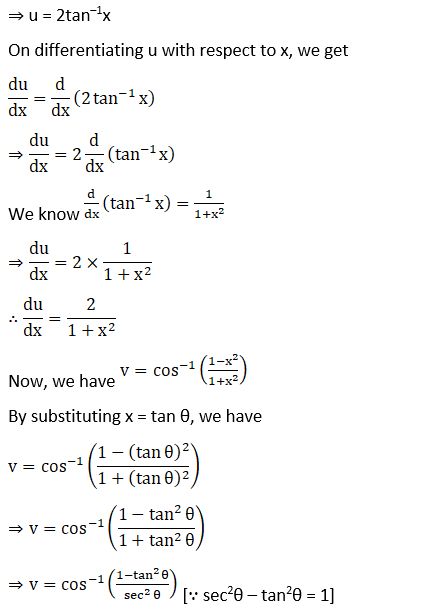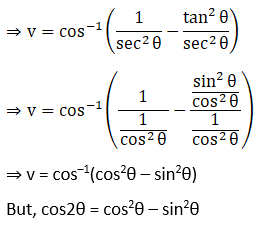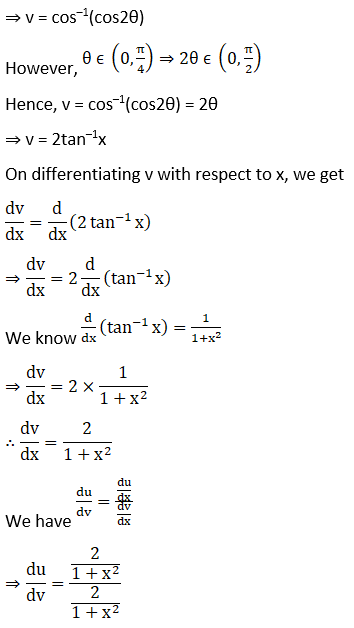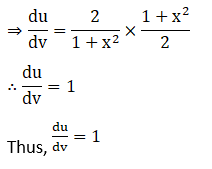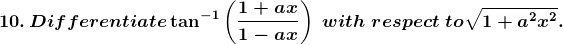Solution: